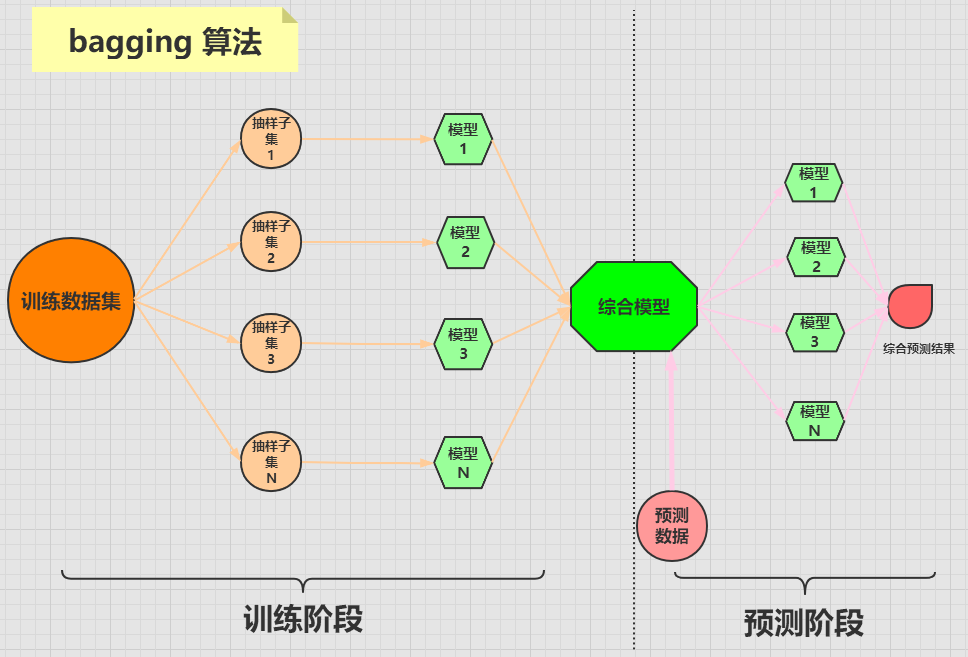### 1，集成算法之 bagging 算法

bagging 算法是将一个原始数据集随机抽样成 N 个新的数据集。然后将这 N 个新的数据集作用于同一个机器学习算法，从而得到 N 个模型，最终集成一个综合模型。

bagging 算法的流程可用下图来表示：### 3，随机森林算法的实现

sklearn 库即实现了随机森林分类树，又实现了随机森林回归树

RandomForestClassifier 类的原型如下：

RandomForestClassifier(n_estimators=100,
criterion='gini', max_depth=None,
min_samples_split=2, min_samples_leaf=1,
min_weight_fraction_leaf=0.0,
max_features='auto', max_leaf_nodes=None,
min_impurity_decrease=0.0,
min_impurity_split=None,
bootstrap=True, oob_score=False,
n_jobs=None, random_state=None,
verbose=0, warm_start=False,
class_weight=None, ccp_alpha=0.0,
max_samples=None)


• n_estimators：随机森林中决策树的个数，默认为 100。
• criterion：随机森林中决策树的算法，可选的有两种：
• gini：基尼系数，也就是 CART 算法，为默认值。
• entropy：信息熵，也就是 ID3 算法
• max_depth：决策树的最大深度。

RandomForestRegressor 类的原型如下：

RandomForestRegressor(n_estimators=100,
criterion='mse', max_depth=None,
min_samples_split=2, min_samples_leaf=1,
min_weight_fraction_leaf=0.0,
max_features='auto', max_leaf_nodes=None,
min_impurity_decrease=0.0,
min_impurity_split=None,
bootstrap=True, oob_score=False,
n_jobs=None, random_state=None,
verbose=0, warm_start=False,
ccp_alpha=0.0, max_samples=None)


• mse：表示均方误差算法，为默认值。
• mae：表示平均误差算法

### 4，随机森林算法的使用

from sklearn.datasets import load_iris

features = iris.data	# 获取特征集
labels = iris.target    # 获取目标集


from sklearn.model_selection import train_test_split

train_features, test_features, train_labels, test_labels =
train_test_split(features, labels, test_size=0.33, random_state=0)


from sklearn.ensemble import RandomForestClassifier

# 这里均使用默认参数
rfc = RandomForestClassifier()

# 训练模型
rfc.fit(train_features, train_labels)


estimators_ 属性中存储了训练出来的所有的子分类器，来看下子分类器的个数：

>>> len(rfc.estimators_)
100


test_predict = rfc.predict(test_features)


>>> from sklearn.metrics import accuracy_score
>>> accuracy_score(test_labels, test_predict)
0.96


### 5，模型参数调优

sklearn 库中有一个 GridSearchCV 类，可以帮助我们进行参数调优。

GridSearchCV(estimator,
param_grid, scoring=None,
n_jobs=None, refit=True,
cv=None, verbose=0,
pre_dispatch='2*n_jobs',
error_score=nan,
return_train_score=False)


• estimator：表示为哪种机器学习算法进行调优，比如随机森林，决策树，SVM 等。
• param_grid：要优化的参数及取值，输入的形式是字典或列表
• scoring：准确度的评价标准。
• cv：交叉验证的折数，默认是三折交叉验证。

from sklearn.datasets import load_iris



from sklearn.ensemble import RandomForestClassifier

rfc = RandomForestClassifier()


param = {"n_estimators": range(1,11)}


from sklearn.model_selection import GridSearchCV

gs = GridSearchCV(estimator=rfc, param_grid=param)

# 对iris数据集进行分类
gs.fit(iris.data, iris.target)


>>> gs.best_score_
0.9666666666666668
>>> gs.best_params_
{'n_estimators': 7}


### 6，总结

（本节完。）